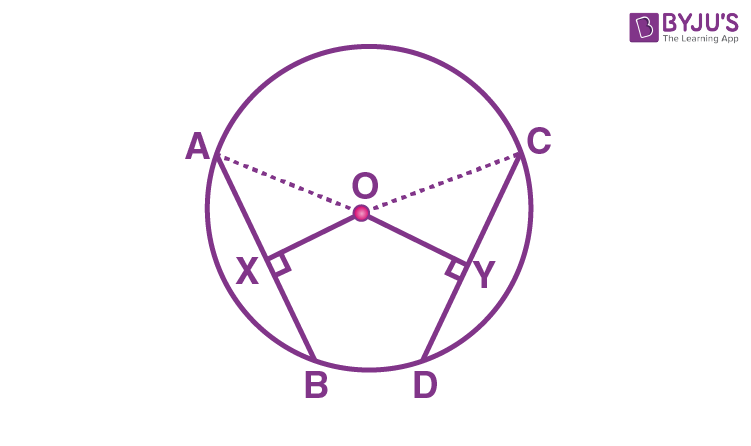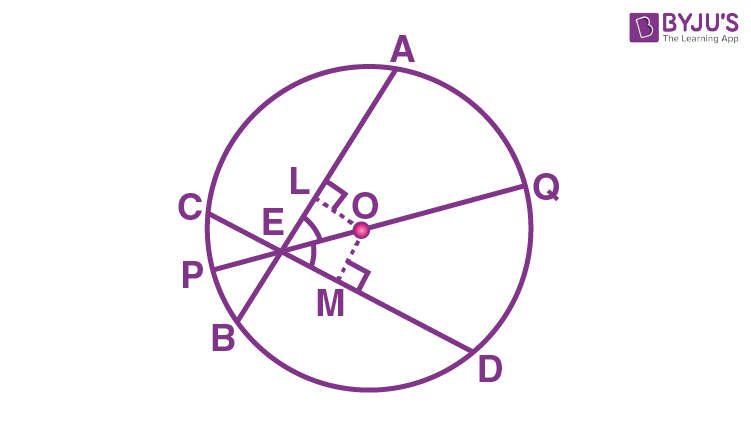# Equal Chords and Their Distances from the Centre

In Mathematics, a chord is the line segment which joins two points on the circumference of a circle. In general, a circle can have infinitely many chords. The distance of the line from a point is defined as the perpendicular distance from a point to a line. If you draw infinite chords to a circle, the longer chord is close to the centre of the circle, than the smaller chord of a circle. In this article, we will discuss the theorem and proof related to the equal chords and their distance from the centre and also its converse theorem in detail.

## Equal Chords and their Distance from the Centre – Theorem and Proof

### Theorem:

Equal chords of a circle (or of congruent circles) are equidistant from the centre (or centres).

### Proof:

Consider a circle with “O”.AB and CD are the equal chords of a circle.

(i.e) AB = CD

The line OX is perpendicular to the chord AB and OY is perpendicular to the chord CD.

We have to prove OX = OY.

Also, the line OX is perpendicular to AB.

As the perpendicular from the centre of the circle to a chord, bisects the chord, we can write it as

AX = BX = AB/2 …(1)

Similarly, the line OY is perpendicular to CD,

CY = DY = CD/2 ….(2) [Since, the perpendicular from the centre of the circle to a chord, bisects the chord)

Given that, AB = CD

AB/2 = CD/2

Therefore, AX = CY …(3) [Using (1) and (2)]

Now, by using the triangles AOX and COY,

∠ OXA = ∠ OYC (Both are 90°)

AX = CY (From equation (3))

From the RHS rule, we can write it as

∆ AOX ≅ ∆ COY

Hence, we can conclude that OX = OY (Using CPCT)

Therefore, the theorem “equal chords of a circle (or of congruent circles) are equidistant from the centre (or centres)”, is proved.

### Converse of this Theorem

The converse of the above-mentioned theorem is “chords equidistant from the centre of a circle are equal in length”.

### Example

Question:

Two intersecting chords of a circle make equal angles with the diameter that passes through their point of intersection. Prove that the chords are equal.

Solution:

Given that AB and CD are the two chords of a circle with centre “O” and it intersects at the point “E” as shown in the figure.PQ is the diameter of a circle (longest chord of a circle), that passes through the point of intersection E, such that it makes an angle ∠ AEQ = ∠ DEQ.

Now, we need to prove that AB = CD.

Now, draw the perpendicular OL to the chord AB and the perpendicular line OM to the chord CD.

By using the angle sum property of a triangle, we can write it as:

∠ LOE = 180° – 90° – ∠ LEO

∠ LOE = 90° – ∠ LEO

= 90° – ∠ AEQ = 90° – ∠ DEQ

= 90° – ∠ MEO = ∠ MOE

Therefore,

∠ LOE = ∠ MOE

From the triangles, OLE and OME, we observe that

∠ LOE = ∠ MOE

Also, ∠ LEO = ∠ MEO

EO = EO (Common side)

Therefore, we will say that the triangles OLE and OME are congruent to each other.

(i.e) ∆ OLE ≅ ∆ OME

Thus, by using the CPCT rule,

OL = OM

By using equal chords and their distance from the centre theorem, we can say,

AB = CD.

So, the chords AB and CD are equal.

Hence, proved.

### Practice Problems

Solve the following problems.

1. Find the length of the common chord, if two circles of radii 5 cm and 3 cm intersect at two points and the distance between the centres is 4 cm.
2. The two equal chords of a circle intersect within a circle. Prove that the segments of one chord are equal to the corresponding segments of the other chord.

Stay tuned with BYJU’S – The Learning App and also learn many Maths-related concepts and Maths theorems easily by exploring exciting videos.